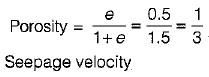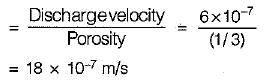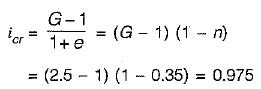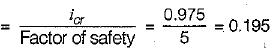Courses

# Seepage Analysis - 2

## 8 Questions MCQ Test Mock Test Series for Civil Engineering (CE) GATE 2020 | Seepage Analysis - 2

Description
This mock test of Seepage Analysis - 2 for Civil Engineering (CE) helps you for every Civil Engineering (CE) entrance exam. This contains 8 Multiple Choice Questions for Civil Engineering (CE) Seepage Analysis - 2 (mcq) to study with solutions a complete question bank. The solved questions answers in this Seepage Analysis - 2 quiz give you a good mix of easy questions and tough questions. Civil Engineering (CE) students definitely take this Seepage Analysis - 2 exercise for a better result in the exam. You can find other Seepage Analysis - 2 extra questions, long questions & short questions for Civil Engineering (CE) on EduRev as well by searching above.
QUESTION: 1

### Darcy’s law for flow through soil is valid if Reynold's number is less than

Solution:

Darcy's law is valid if the flow through soil is laminar and Reynolds number is less than 1.

QUESTION: 2

### Consider the following statements: 1. Seepage force is applied by flowing water to the soil skeleton through frictional drag. 2. The magnitude of seepage force per unit volume of soil at any point is equal to Yw/i, where Yw is the unit weight of water and i is the hydraulic gradient at that point. 3. In a soil mass subjected to upward flow of water, quick sand condition develops when pore pressure is equal to the total stress Which of these statements is/are correct?

Solution:

Seepage force is due to frictional drag and its magnitude is given by iYw per unit volume of soil. For quick condition during upward flow.
Effective stress = total stress - pore pressure So statement 1 and 3 is correct.

*Multiple options can be correct
QUESTION: 3

### Which one of the following explains the flow condition occurring within a soil when its effective pressure is reduced to zero?

Solution:

When the seepage of water is upward in a soil layer so much that seepage force decreases the inter particle forces and effective stress is reduced to zero, quick sand condition occurs. Liquefaction of sand is also a phenomenon when effective stress of soil is reduced to zero, it usually occurs when sand deposit is shaken due to an earthquake or any other oscillatory load which increases the pore water pressure.

QUESTION: 4

A soil has a discharge velocity of 6 x 10-7 m/s and a void ratio of 0.5. Its seepage velocity is

Solution:QUESTION: 5

For an anisotropic soil, permeabilities in x and y directions are Kx and Ky respectively in a two dimensional flow. The effective permeability Keq for the soil is given by

Solution:
QUESTION: 6

To provide safety against piping failure, with a factor of safety of 5, what should be the maximum permissible exit gradient for soil with specific gravity of 2.5 and porosity of 0.35?

Solution:∴ Maximum permissible exit gradient,QUESTION: 7

During seepage through an earth mass, the direction of seepage is

Solution:
QUESTION: 8

The upstream slope of an earth dam under steady seepage condition is

Solution: